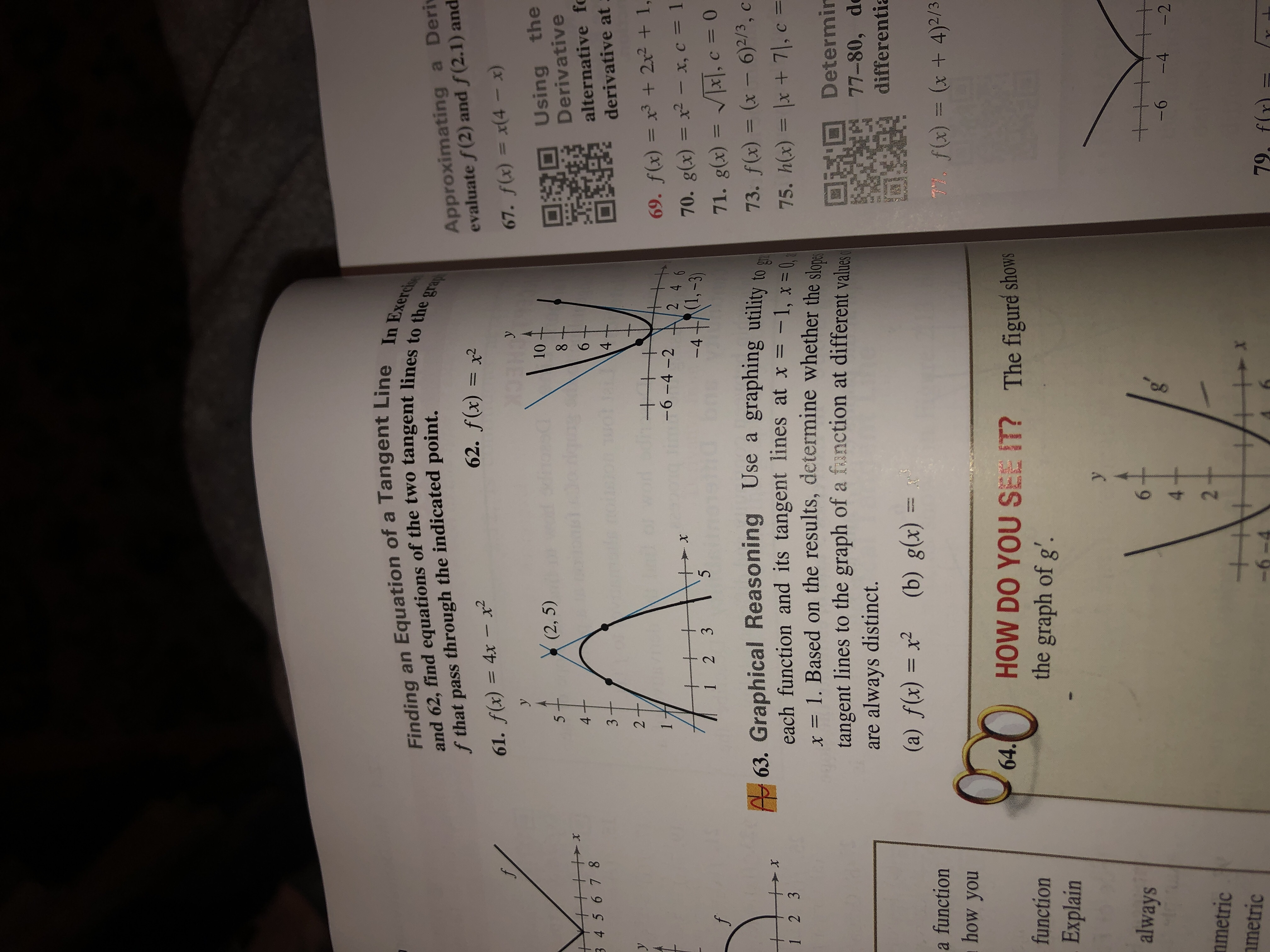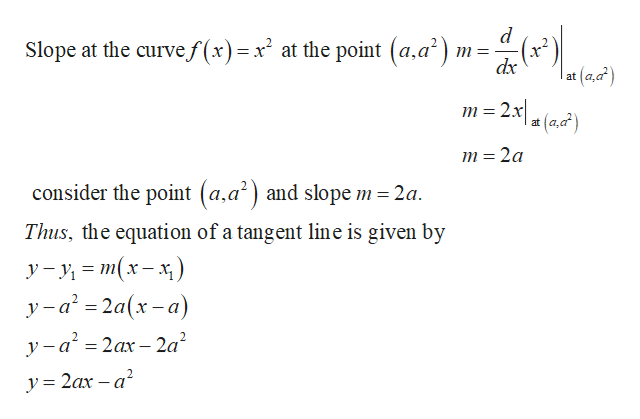# Finding an Equation of a Tangent Line In Exercisand 62, find equations of the two tangent lines to the grapApproximating a Derievaluate f(2) and f(2.1) andf that pass through the indicated point.62. f(x) = x261. f(x) = 4x- x267. f(x) = x(4 - xyуUsing theDerivative10(2, 5)864alternative fe3 45 6 784derivative at3+wwwe2y69. f(x) = x3 + 2x2 + 1,-6-4 -22 4 670. g(x) = x2 - x, c = 1-XRI,-3)-451 2 371. g(x) = x, c = 0fA 63. Graphical Reasoning Use aeach function and its tangent lines at x = -1, x =x =1. Based on the results, determine whether the slanetangent lines to the graph of a fanction at different valuesalways distinct.graphing utility to73. f(x) = (x - 6)2/3, cx75. h(x) = lx +7|, c =C1 2 3Determir77-80, dearedifferentia(b) g(x) =(a) f(x) xTY f(x)(x + 4)2/3a functionhow youHOW DO YOU SEE IT? The figuré showsthe graph of g'.64.functionExplain+talways-6-4-242+nmetricmetric79, f(r-6-4ob151

Question
7 views

61help_outlineImage TranscriptioncloseFinding an Equation of a Tangent Line In Exercis and 62, find equations of the two tangent lines to the grap Approximating a Deri evaluate f(2) and f(2.1) and f that pass through the indicated point. 62. f(x) = x2 61. f(x) = 4x- x2 67. f(x) = x(4 - x y у Using the Derivative 10 (2, 5) 8 6 4 alternative fe 3 45 6 78 4 derivative at 3+ wwwe 2 y 69. f(x) = x3 + 2x2 + 1, -6-4 -2 2 4 6 70. g(x) = x2 - x, c = 1 -X RI,-3) -4 5 1 2 3 71. g(x) = x, c = 0 f A 63. Graphical Reasoning Use a each function and its tangent lines at x = -1, x = x =1. Based on the results, determine whether the slane tangent lines to the graph of a fanction at different values always distinct. graphing utility to 73. f(x) = (x - 6)2/3, c x 75. h(x) = lx +7|, c = C 1 2 3 Determir 77-80, de are differentia (b) g(x) = (a) f(x) x TY f(x)(x + 4)2/3 a function how you HOW DO YOU SEE IT? The figuré shows the graph of g'. 64. function Explain +t always -6 -4 -2 4 2+ nmetric metric 79, f(r -6-4 ob 151 fullscreen
check_circle

Step 1

Let (a, f(a)) be a point on the curve f(x) = x2 . now find the slope of the curve at the...help_outlineImage Transcriptionclosed Slope at the curve f (x)=x at the point (a.a m= - dx at m= 2x\ at (a,a m 2a consider the point (a.a) and slope m = 2a Thus, the equation of a tangent line is given by y- (x-x y-a2a(x-a) ya2ax-2a _ = y 2ax -a fullscreen

### Want to see the full answer?

See Solution

#### Want to see this answer and more?

Solutions are written by subject experts who are available 24/7. Questions are typically answered within 1 hour.*

See Solution
*Response times may vary by subject and question.
Tagged in

### Derivative